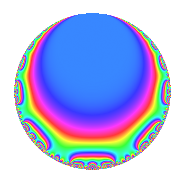# Properties

 Label 672.2.a.jLevel 672 Weight 2 Character orbit 672.a Self dual Yes Analytic conductor 5.366 Analytic rank 0 Dimension 2 CM No Inner twists 1

# Related objects

## Newspace parameters

 Level: $$N$$ = $$672 = 2^{5} \cdot 3 \cdot 7$$ Weight: $$k$$ = $$2$$ Character orbit: $$[\chi]$$ = 672.a (trivial)

## Newform invariants

 Self dual: Yes Analytic conductor: $$5.36594701583$$ Analytic rank: $$0$$ Dimension: $$2$$ Coefficient field: $$\Q(\sqrt{3})$$ Coefficient ring: $$\Z[a_1, \ldots, a_{5}]$$ Coefficient ring index: $$2$$ Fricke sign: $$-1$$ Sato-Tate group: $\mathrm{SU}(2)$

## $q$-expansion

Coefficients of the $$q$$-expansion are expressed in terms of $$\beta = \sqrt{3}$$. We also show the integral $$q$$-expansion of the trace form.

 $$f(q)$$ $$=$$ $$q + q^{3} + 2 \beta q^{5} - q^{7} + q^{9} +O(q^{10})$$ $$q + q^{3} + 2 \beta q^{5} - q^{7} + q^{9} + ( 2 - 2 \beta ) q^{11} + 2 q^{13} + 2 \beta q^{15} + ( 4 - 2 \beta ) q^{17} + 4 \beta q^{19} - q^{21} + ( 2 - 2 \beta ) q^{23} + 7 q^{25} + q^{27} + ( 2 - 4 \beta ) q^{29} + ( -4 - 4 \beta ) q^{31} + ( 2 - 2 \beta ) q^{33} -2 \beta q^{35} -2 q^{37} + 2 q^{39} + ( 8 + 2 \beta ) q^{41} -8 q^{43} + 2 \beta q^{45} + ( 4 + 4 \beta ) q^{47} + q^{49} + ( 4 - 2 \beta ) q^{51} -2 q^{53} + ( -12 + 4 \beta ) q^{55} + 4 \beta q^{57} + ( -8 + 4 \beta ) q^{59} + ( -2 - 4 \beta ) q^{61} - q^{63} + 4 \beta q^{65} + ( 4 - 4 \beta ) q^{67} + ( 2 - 2 \beta ) q^{69} + ( 6 + 2 \beta ) q^{71} + ( 6 + 4 \beta ) q^{73} + 7 q^{75} + ( -2 + 2 \beta ) q^{77} + ( -4 - 4 \beta ) q^{79} + q^{81} -4 q^{83} + ( -12 + 8 \beta ) q^{85} + ( 2 - 4 \beta ) q^{87} + 2 \beta q^{89} -2 q^{91} + ( -4 - 4 \beta ) q^{93} + 24 q^{95} + ( -2 - 4 \beta ) q^{97} + ( 2 - 2 \beta ) q^{99} +O(q^{100})$$ $$\operatorname{Tr}(f)(q)$$ $$=$$ $$2q + 2q^{3} - 2q^{7} + 2q^{9} + O(q^{10})$$ $$2q + 2q^{3} - 2q^{7} + 2q^{9} + 4q^{11} + 4q^{13} + 8q^{17} - 2q^{21} + 4q^{23} + 14q^{25} + 2q^{27} + 4q^{29} - 8q^{31} + 4q^{33} - 4q^{37} + 4q^{39} + 16q^{41} - 16q^{43} + 8q^{47} + 2q^{49} + 8q^{51} - 4q^{53} - 24q^{55} - 16q^{59} - 4q^{61} - 2q^{63} + 8q^{67} + 4q^{69} + 12q^{71} + 12q^{73} + 14q^{75} - 4q^{77} - 8q^{79} + 2q^{81} - 8q^{83} - 24q^{85} + 4q^{87} - 4q^{91} - 8q^{93} + 48q^{95} - 4q^{97} + 4q^{99} + O(q^{100})$$

## Embeddings

For each embedding $$\iota_m$$ of the coefficient field, the values $$\iota_m(a_n)$$ are shown below.

For more information on an embedded modular form you can click on its label.

Label $$\iota_m(\nu)$$ $$a_{2}$$ $$a_{3}$$ $$a_{4}$$ $$a_{5}$$ $$a_{6}$$ $$a_{7}$$ $$a_{8}$$ $$a_{9}$$ $$a_{10}$$
1.1
 −1.73205 1.73205
0 1.00000 0 −3.46410 0 −1.00000 0 1.00000 0
1.2 0 1.00000 0 3.46410 0 −1.00000 0 1.00000 0
 $$n$$: e.g. 2-40 or 990-1000 Significant digits: Format: Complex embeddings Normalized embeddings Satake parameters Satake angles

## Inner twists

This newform does not admit any (nontrivial) inner twists.

## Atkin-Lehner signs

$$p$$ Sign
$$2$$ $$1$$
$$3$$ $$-1$$
$$7$$ $$1$$

## Hecke kernels

This newform can be constructed as the intersection of the kernels of the following linear operators acting on $$S_{2}^{\mathrm{new}}(\Gamma_0(672))$$:

 $$T_{5}^{2} - 12$$ $$T_{11}^{2} - 4 T_{11} - 8$$ $$T_{19}^{2} - 48$$